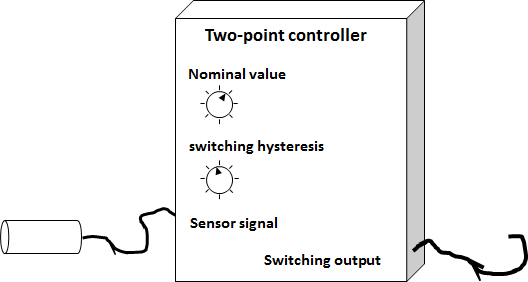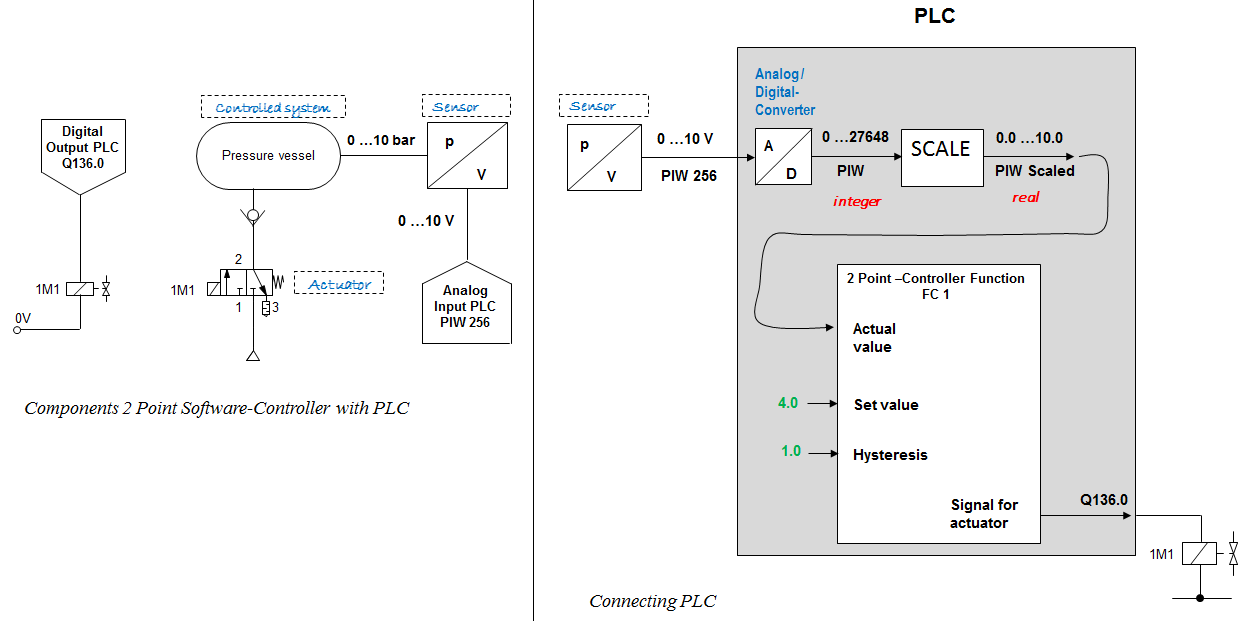# Two-point controller

## Two-point-controller - how it works

Two-point controllers, also known as On-Off-Controllers or Binary Controllers are used for slow control systems. Here, the actual value changes only slowly. Examples for this: temperature control, level control, compressed air control, etc.

The following animation illustrates the function of a two-point controller at the example of a compressed air control. The air tank represents a PT1-controlled system.

The control behavior of a two-point controller in combination with a PTn- system looks quite different due to the overshoot of the controlled variable. As an example, a zinc bath is used. The heating resistors represent the first energy storage, the zinc the second energy storage. Therefor the zinc bath can be classified as a PT2 system.

The parameter of a two-point controller is the setpoint and the switching hysteresis. The sensor signal is an input signal but not a parameter.Two-Point-Controller - Parameter and connections

The switching and control behavior of a 2-point controller is further clarified by the static y(x) characteristic curve. By the way, this results in the symbol of this controller.2-Point-Controller - static curve

Since the time axis t is the common axis, both diagrams y(t) and x(t) can be combined to form the static y(x) characteristic diagram.

### Work order: Programming of a software Two-Point Controller with PLC

First you make an overview of the necessary components and how they may be connected to the PLC. In the following a Siemens PLC, more precisely CPU314 is used. The addresses of the inputs and outputs can also be modified via the HW configuration.Structure - Two-Point-Control with PLC

If all the necessary components are available and the required PLC inputs or outputs are not yet connected, you cab begin to plan the program. In order to get at an algorithm at all, you should know how determine the Turn-on and Turn-off points.Two-Point Controller - Switch-On and Switch-Off point

The flowchart and the structogram (also referred to as the Nassi-Schneider diagram) are used in particular to describe the program sequence:Programming a Two-Point Controller